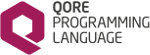Qore Programming Language Reference Manual  0.9.16
RangeIterator helper functions

RangeIterator helper functions allow to use RangeItartor in easier usage in loop statements. More...

## Functions

RangeIterator Qore::xrange (int start, int stop, int step=1, auto val)
Returns a RangeIterator containing an arithmetic progression of integers. More...

RangeIterator Qore::xrange (int stop)
Returns a RangeIterator containing an arithmetic progression of integers with start = 0 and step = 1. More...

## Detailed Description

RangeIterator helper functions allow to use RangeItartor in easier usage in loop statements.

Example
foreach int i in(xrange(5))
printf("i=%d\n", i);
# resulting in:
# i=0
# i=1
# i=2
# i=3
# i=4
# i=5

## ◆ xrange() [1/2]

 RangeIterator Qore::xrange ( int start, int stop, int step = `1`, auto val )

Returns a RangeIterator containing an arithmetic progression of integers.

Code Flags:
RET_VALUE_ONLY
Example:
xrange(2, 5); # 2, 3, 4, 5
xrange(2, -2); # 2, 1, 0, -1, -2
xrange(1, 10, 5); # 1, 6
xrange(0, 10, 5); # 0, 5, 10
xrange(-10, 10, 5); # -10, -5, 0, 5, 10
xrange(10, -10, 5); # 10, 5, 0, -5, -10
Parameters
 start the initial value stop the final value step the step; the default is 1; must be greater than 0; the function throws a `RANGE-ERROR` exception when this argument is < 1 val an optional value to be returned instead of the default integer value
Return values
 Returns a RangeIterator containing an arithmetic progression of integers.
Exceptions
 RANGEITERATOR-ERROR this exception is thrown if `step` < 1
range
Note
the main difference between range() and xrange() is that range returns a real list and xrange() returns a RangeIterator
Since
• Qore 0.8.6
• Qore 0.8.11.1 this function takes the optional val argument
• Qore 0.9.5 does not include the upper limit in the range unless %broken-range is set

## ◆ xrange() [2/2]

 RangeIterator Qore::xrange ( int stop )

Returns a RangeIterator containing an arithmetic progression of integers with start = 0 and step = 1.

This is an overloaded version of xrange(int, int, int) meaning `xrange`(0, `stop`, 1)

Code Flags:
CONSTANT
Example:
xrange(1); # 0, 1
xrange(-3); # 0, -1, -2, -3
Parameters
 stop the final value
Return values
 Returns a RangeIterator containing an arithmetic progression of integers with start = 0 and step = 1.